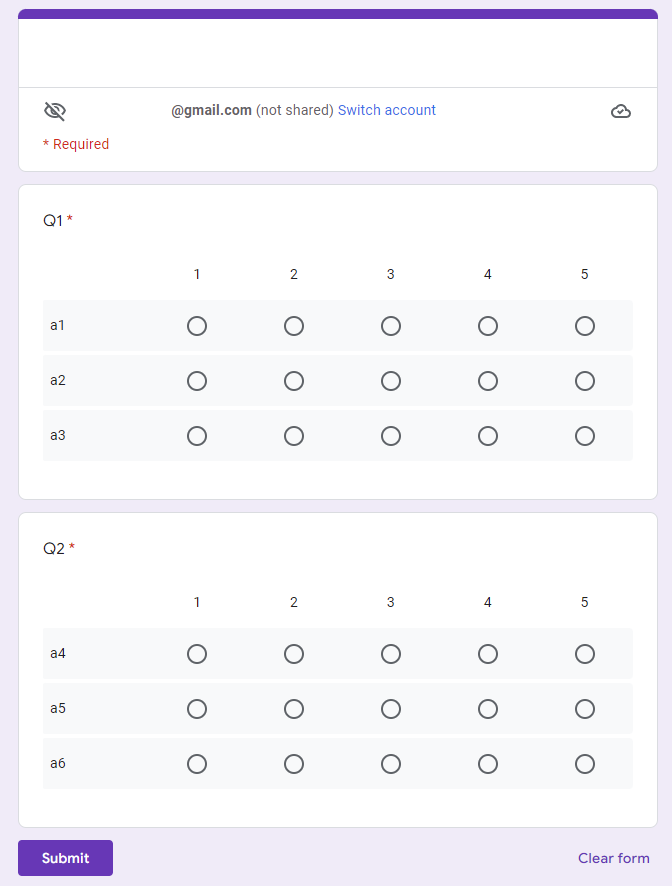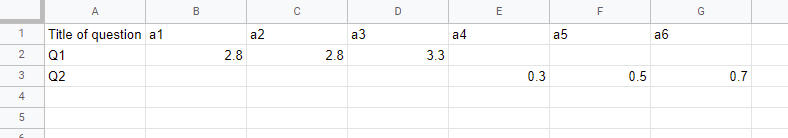# Analyzing Responses from Grid Items of Google Form using Google Apps Script

Gists

This is a sample script for analyzing the responses from Grid Items of Google Form using Google Apps Script.

In this sample situation, all responses are retrieved from Grid Items of Google Form, and the average values of each row of each question are calculated. And, the result is put on the Spreadsheet.

## Sample situation

The sample Google Form is as follows.The sample output is as follows.The average values of each row of Grid Items of each question are calculated and put to the Spreadsheet.

## Sample script

function myFunction() {
const sheetName = "Sheet1"; // Please set the sheet name.

// Retrieve responses from the grid items.
const form = FormApp.openById(formId);
const formResponses = form.getResponses();
const obj = formResponses.reduce(
(o, e) => {
e.getItemResponses().forEach((f) => {
const item = f.getItem();
if (item.getType() == FormApp.ItemType.GRID) {
const title = item.getTitle();
o.res[title] = o.res[title] || {};
const response = f.getResponse();
const rows = item.asGridItem().getRows();
rows.forEach((g, k) => {
const v = Number(response[k]) || 0;
o.res[title][g] = o.res[title][g] ? o.res[title][g] + v : v;
});
}
});
return o;
},
{ res: {}, head: [] }
);

// Create an array for putting the values to Spreadsheet.
const len = formResponses.length;
const values = [
...Object.entries(obj.res).map(([k1, v1]) => [
k1,
...header.map((h) => (v1[h] ? v1[h] / len : null)),
]),
];

// Put the values to Spreadsheet.